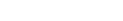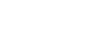Common Derivatives on the AP Calc Exam

You know you’ll have to know your derivatives inside and out in order to score high on the AP Calculus exam. But what are the most common derivatives you’ll see on the test? In this short article, we’ll let you in on the secret!

Know the Basics

First of all, it’s very important to have the basics down. That includes: the Power Rule, Product Rule, Quotient Rule, and Chain Rule, among others.

Don’t forget about those special functions either. You’ll have to memorize the derivative rules for trig, exponential, and logarithmic functions.Derivatives of trigonometric, exponential, and logarithmic functions

Less common, but no less important are the rules for inverse trig functions.Derivatives of inverse trigonometric functions

Common Derivatives

Now let’s take a look at a few problems involving common derivatives that are modeled after actual AP Calculus problems.

Problem 1

Find The tangent line to the curve f(x) = x4 + 3x – 10 at the point (1, -6).

Solution

To find a tangent line, first take the derivative. Here, we have to use the Power Rule and Sum/Difference Rule.

f '(x) = 4x3 + 3

Then you can find the slope and the equation of the tangent line.

Slope at x = 1: f '(1) = 4(1)3 + 3 = 7

y – (-6) = 7(x – 1)

y = 7x – 13.

Problem 2

The position of a particle moving along the x-axis at time t is x(t) = sin( cos( 4t ) ), for 0 ≤ t ≤ π. Find
the velocity at time t = π/8.

Solution

Find velocity by taking the derivative of the position function. Be careful — we need two applications of the Chain Rule for this one!

v(t) = x '(t) = cos( cos( 4t ) ) ( -sin( 4t ) ) (4)

At time t = π/8, the velocity is equal to:

v(π/8) = cos( cos( 4(π/8) ) ) ( -sin( 4(π/8) ) ) (4) = cos( cos( π/2 ) ) ( -sin( π/2 ) ) (4)

= cos(0)(-1)(4) = (1)(-1)(4) = -4.

Problem 3

Find all inflection points of the curve defined by.

Solution

You can find inflection points by taking the second derivative. Of course, you must take the first derivative first. Don’t forget to rewrite your radical as a power and use Chain Rule.Use Quotient Rule to help find the second derivative.After simplification, we find that the second derivative is never equal to 0 and never undefined. Therefore this function has no inflection points.

Problem 4Solution

You’ll need both the Product and Chain Rules for this one.Problem 5

Suppose g(x) = e4x + 5. At what value of x is the slope of the tangent line to g(x) equal to 3?

Solution

Again, the slope of the tangent line is equal to a derivative value.

g '(x) = 4e4x

Set g '(x) = 3 and solve:

4e4x = 3

e4x = 3/4

4x = ln(3/4)

x = ln(3/4) / 4 ≈ -0.0719

Summary

The five problems above represent just a small sampling of what you’ll find on an AP Calculus AB or BC exam. Nevertheless, the majority of problems involving derivatives do tend to fall into these basic formats.

• Know your basic rules, especially the Chain Rule.
• Memorize the derivatives of the special functions.
• And above all, know which tools apply in each situation.

Good luck on the exam!

Improve your SAT or ACT score, guaranteed. Start your 1 Week Free Trial of Magoosh SAT Prep or your 1 Week Free Trial of Magoosh ACT Prep today!Author

•Shaun earned his Ph. D. in mathematics from The Ohio State University in 2008 (Go Bucks!!). He received his BA in Mathematics with a minor in computer science from Oberlin College in 2002. In addition, Shaun earned a B. Mus. from the Oberlin Conservatory in the same year, with a major in music composition. Shaun still loves music -- almost as much as math! -- and he (thinks he) can play piano, guitar, and bass. Shaun has taught and tutored students in mathematics for about a decade, and hopes his experience can help you to succeed!